Enhancing parameter precision of optimal quantum estimation by quantum screening
Physical and Photoelectric Science Department, Guangdong Ocean University, Zhanjiang 524088, China
Department of Electronic and Communication Engineering, Changsha University, Changsha 410003, China

† Corresponding author. E-mail: guoxuyan2007@163.com

Project supported by the National Natural Science Foundation of China (Grant No. 11374096), the Natural Science Foundation of Guangdong Province, China (Grants No. 2015A030310354), and the Project of Enhancing School with Innovation of Guangdong Ocean University (Grants Nos. GDOU2014050251 and GDOU2014050252).

Abstract
Abstract

We propose a scheme of quantum screening to enhance the parameter-estimation precision in open quantum systems by means of the dynamics of quantum Fisher information. The principle of quantum screening is based on an auxiliary system to inhibit the decoherence processes and erase the excited state to the ground state. By comparing the case without quantum screening, the results show that the dynamics of quantum Fisher information with quantum screening has a larger value during the evolution processes.

1. Introduction

Quantum metrology, which investigates the ultra-precise estimation of parameters, has been widely applied in quantum precise measurement, quantum frequency standards, quantum clock synchronization, single photon detections,[8,9] and so on. The main task of quantum parameter estimation is to determine the precision of a physical parameter in quantum systems. Quantum Fisher information (QFI), which can be used as measurement of probability distribution on the space of the density operators, becomes a key quantity in quantum estimation theory. More recently, enhancing parameter precision of optimal quantum estimation by direct quantum feedback was reported. The authors found that the QFI could be obviously enhanced by quantum feedback. Besides, the estimation of the quantum channel parameter for a two-qubit system with each qubit independently interacting with its Markovian environment has also been investigated. The authors studied the dynamics of QFI with respect to the decoherence rate and the interaction strength. According to the quantum Cramér–Rao (QCR) inequality, the value of QFI provides an upper bound on the parameter precision. Therefore, the larger QFI indicates the higher precision of the estimated parameter. At the same time, for the properties of monotonicity and convexity, QFI closely connects with other quantities such as quantum entanglement,[22,23] spin squeezing, and non-Markovian effect.[14,18]

However, any realistic quantum system is open since the unavoidable interaction with its environment induced the decoherence belonging to the coupled systems. According to the styles of information flow, the time evolution of the quantum system can be divided into two classes: One is a Markovian process, which means the coupling of the system and its environment is weak so that one can address the model with Born–Markov approximation. The main characteristic of the Markovian process is that the information flow is monotonic. The other is a non-Markovian process,[14,18] which has a vibration revival effect because of the strong coupling between the system and its environment.

In this work, we propose another alternative method, quantum screening, to enhance the QFI of a qubit coupling with the zero temperature reservoir in a Markovian process. In the electromagnetic field theory, an electronic device is closed in the metal casing, which is connected with the zero-ground potential, that can be screened from the disturbing effects of the external electromagnetic field. Inspired by the zero-ground screening scheme, we utilize an auxiliary system to erase the excited state to the ground state. We examine the parameter estimation of a dissipative qubit system by the quantum screening with the help of QFI, and demonstrate that the number of erasing events is proportional to the precision of the parameter. The results show that QFI can be enhanced by quantum screening. The effects of Rabi frequency and the number of erasing events on QFI are also considered. Besides, a reasonable physical explanation is given.

This paper is organized as follows: In Section 2, we introduce the basic knowledge of QFI and give the expressions of QFI with pure and mixed states. In Section 3, we discuss the main idea of the quantum screening effect on QFI of a dissipative qubit under critical and non-critical damping cases. Finally, we briefly summarize the paper in Section 4.

2. Quantum Fisher information

Firstly we review some basic conceptions of parameter estimation theorem and QFI which will be used in this paper. As we know, the main goal of parameter estimation is to acquire the best observable. Considering a quantum state ρ(θ), we need to estimate the observablewhich is called the unbiased estimator. According to the QCR theory, we can quantify the precision of a parameter with QFI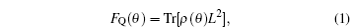where L is the so-called symmetric logarithmic derivation satisfying the equation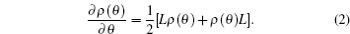The QCR inequality gives a limitation by a bound to the variance of parameter estimation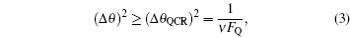where (Δθ)2 represents the mean square error of θ, and ν denotes the number of repeated independent experiments. The larger FQ implies the smaller variance. In principle, equation (3) offers the best possible precision estimation of the value of phase. We use the approach of QFI to compute the precision of parameter estimation for quantum screening dynamics in this paper.

The screening interferometric phase estimation method is plotted in Fig. 1. To estimate the unknown parameter θ as precisely as possible, the author in Ref.  pointed out that the optimal input state may be chosen as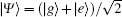which maximizes the QFI of the output state |Ψ〉 after the action of phase gate U(ϕ). Under this condition, the QFI of the output state reaches its maximum value and the single-qubit phase gate operation is given by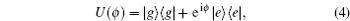where θ = ϕ is the inference parameter. After some algebra, we can give the expressions of QFI for pure and mixed states, respectively. Namely, the QFI of a pure state is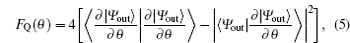the QFI of a mixed state is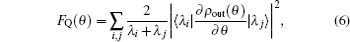where λi and |λi〉 are the eigenvalues and eigenvectors of ρout.

 Figure Option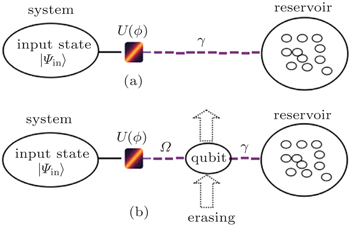Fig. 1. The diagram of screening method: (a) interaction between the qubit and reservoir without screening, (b) the screening method of the interaction.
3. Enhancing the QFI by quantum screening
3.1. Without quantum screening

We first study the dynamics of QFI without screening as shown in Fig. 1(a). We consider a qubit interacting with a single-mode reservoir resonantly, the qubit with Rabi frequency Ω. The time evolution process of the model can be described by the Lindblad equation with Born–Markovian approximation (ħ = 1)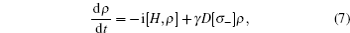where the first term on the right-hand side denotes the Hamiltonian evolution, γ is the spontaneous emission rate and the superoperator is defined as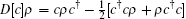. Here we can discard the Hamiltonian term because we are interested in the evolution of the qubit state. The elements of density matrix can be analytically solved under the decoherence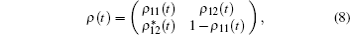where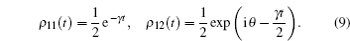Due to the decoherence channel, the output state ρ(t) is a mixed state. According to Eq. (6), the QFI of ρ(t) without quantum screening can be exactly calculated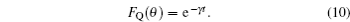3.2. The QFI with screening

Figure 1(b) shows the basic principle of quantum screening, which is based on an auxiliary system to erase the excited state of a qubit to the ground state. In this model, the qubit interacts with the damping single-mode cavity resonantly. In order to overcome the damping process, we employ an auxiliary casing system consisting of the mode of electromagnetic field inside the cavity. The electromagnetic field is initially prepared in the vacuum state. For simplicity, we ignore the off-resonant interaction. The auxiliary screening events divide the evolution time t into N identical intervals that every separated interaction between the qubit and damped cavity lasts a time of T = t/N. With quantum screening, the time evolution of the whole system can be described by the following master equation under Born–Markovian approximation (ħ = 1)where HI = iΩ(aσ+) represents the interaction Hamiltonian. We first discuss the characterization of Rabi frequency Ω in the auxiliary field. By solving Eq. (11), we demonstrate the precision enhancement by means of QFI of a dissipative qubit system with the quantum screening. It is shown that the Rabi frequency of the auxiliary field Ω < γ/4 implies it is in the weak field limit, and such overdamped case exhibits hyperbolic behavior. The strong field limit Ω > γ/4 is the underdamped case with oscillatory behavior; Ω = γ/4 is the case of critical damping. After N erasing events, the analytical solution of the reduced density matrix of the qubit can be obtained with two situations. For non-critical damping (Ωγ/4)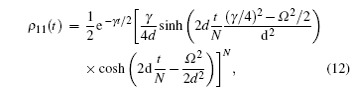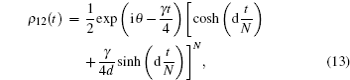where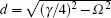. For critical damping (Ω = γ/4)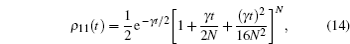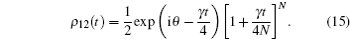Substituting Eqs. (12)–(15) into Eq. (6), it is not hard to calculate the QFI. For non-critical damping (Ωγ/4)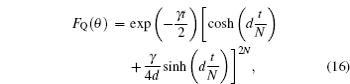and for critical damping (Ω = γ/4)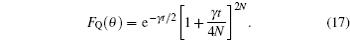Let us now demonstrate that the screening method does enhance the parameter precision for every situation of the constants Ω. The dynamics of QFI for the output state can be compared with respect to non-screening and screening operations for a different order of the Rabi frequency Ω in Fig. 2(a). We plot the QFI as a function of the decay parameter γt and a specific choice of the erasing events N. The dashed line is for non-screening operation, it has an exponential decline, the red line is for overdamped case Ω = γ/8, the green line is for critical damped case Ω = γ/4 and the blue line is for underdamped case Ω = γ/2. The dynamical evolution of QFI without screening appears a sudden change to the minimum value, while the QFI with screening may be delayed during the time evolution for every value of the constant Ω. Hence it is straightforwardly demonstrated that the screening method can enhance the QFI. Moreover, we find another interesting phenomenon that the different values of Ω have different evolution lines. The smaller Ω is, the higher the value of QFI is. Based on the quantum estimation theory, this indicates the higher value of QFI means the optimal precision of estimation is enhancing. In the following, we discuss the factor of erasing events N with a critical damped situation. The evolution of a non-critical damped situation is similar to the critical damped case. Figure 2(b) shows that the evolution of QFI is higher with a larger N, this can be understood intuitively from Eq. (17), which is a monotone increasing function with N. The physical mechanism can be interpreted by the dynamical Zeno effect: The screening operation makes an action of quantum transmission from |Ψ〉|0〉 → |0〉|Ψ〉, which swaps the excited state to the ground state of the cavity. The larger erasing events N corresponds with the bigger probability of swap from excited to ground state, which can decrease the environment decoherence. The interaction between the qubit and auxiliary cavity has a characteristic of non-exponential decoherence. The non-exponential effect is more obvious with a smaller coupling constant Ω, that the phenomenon of sequential arrangement in Fig. 2(a) is easily understood.

 Figure Option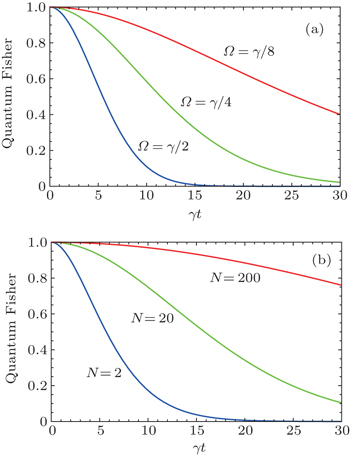Fig. 2. QFI as a function of the damping constant γt. (a) QFI in terms of γt for different values of Rabi frequency Ω with a specific choice of N = 10. (b) QFI in terms of γt for different values of erasing events N in critical damping case Ω = γ/4.
4. Conclusion

In this work, we have studied the QFI of a dissipative qubit system with and without the quantum screening, an auxiliary qubit which is erased from excited state to the ground state. We found that the case with screening can reduce the information, hence enhancing the precision measurement limit. What is more, the Rabi frequency Ω and erasing events N are two meaningful factors to affect the precision; the exact discussion and physical mechanism have been given in context.

Reference
 1 Li S SZhang Z HZhao WLi Z KHuang S L 2015 Chin. Phys. B 24 010601 2 Cai S PDai LNi H 2013 Acta. Phys. Sin. 62 189204 (in Chinese) 3 He ZYao C M 2014 Chin. Phys. B 23 110601 4 Chen H JZhu K D2015Sci. China-Phys. Mech. Astron.5850301 5 Chen Z ZRen X LTang F Q 2013 Chin. Sci. Bull. 58 2622 6 Bollinger J JItano W MWineland D JHeinzen D J 1996 Phys. Rev. A 54 4649 7 Zhang JLong G LDeng ZLiu W ZLu Z H 2004 Phys. Rev. A 70 062322 8 Liang YZeng H P 2014 Sci. China-Phys. Mech. Astron. 57 1218 9 Zhang JItzler M AZbinden H 2015 Light: Science and Applications 4 286 10 Zheng QGe LYao YZhi Q J 2015 Phys. Rev. A 91 033805 11 Berrada K 2015 J. Opt. Soc. Am. B 32 571 12 Lu X MWang X GSun C P 2010 Phys. Rev. A 82 042103 13 Zhong WZhe SMa JWang X GNori F 2013 Phys. Rev. A 87 022337 14 Li Y LXiao XYao Y 2015 Phys. Rev. A 91 052105 15 Liu W FMa JWang X G 2013 J. Phys. A: Math. Theor. 46 045302 16 Tan Q SHuang Y XYin X LKuang L MWang X G 2013 Phys. Rev. A 87 032102 17 Li NLuo S L 2013 Phys. Rev. A 88 014301 18 Berrada K 2013 Phys. Rev. A 88 035806 19 Zhong WLiu JMa JWang X G 2014 Chin. Phys. B 23 060302 20 Zheng QYao YLi Y 2014 Eur. Phys. J. D 68 170 21 Helstrom C W1976Quantum etection and estimation theoryNew YorkAcademic Press 22 Huo W YLong G L 2008 New. J. Phys. 10 013026 23 Fang Y MQin Z ZWang H Let al.2015Sci. China-Phys. Mech. Astron.5864201 24 Nielsen M AChuang I L2000Quantum Computation and Quantum InformatinCambridgeCambridge University Press 25 Filip R 2003 Phys. Rev. A 67 014308 26 Mazhar A 2010 J. Pyhs. B: At. Mol. Opt. Phys. 43 045504 27 Luis APerian J 1996 Phys. Rev. Lett. 76 4340